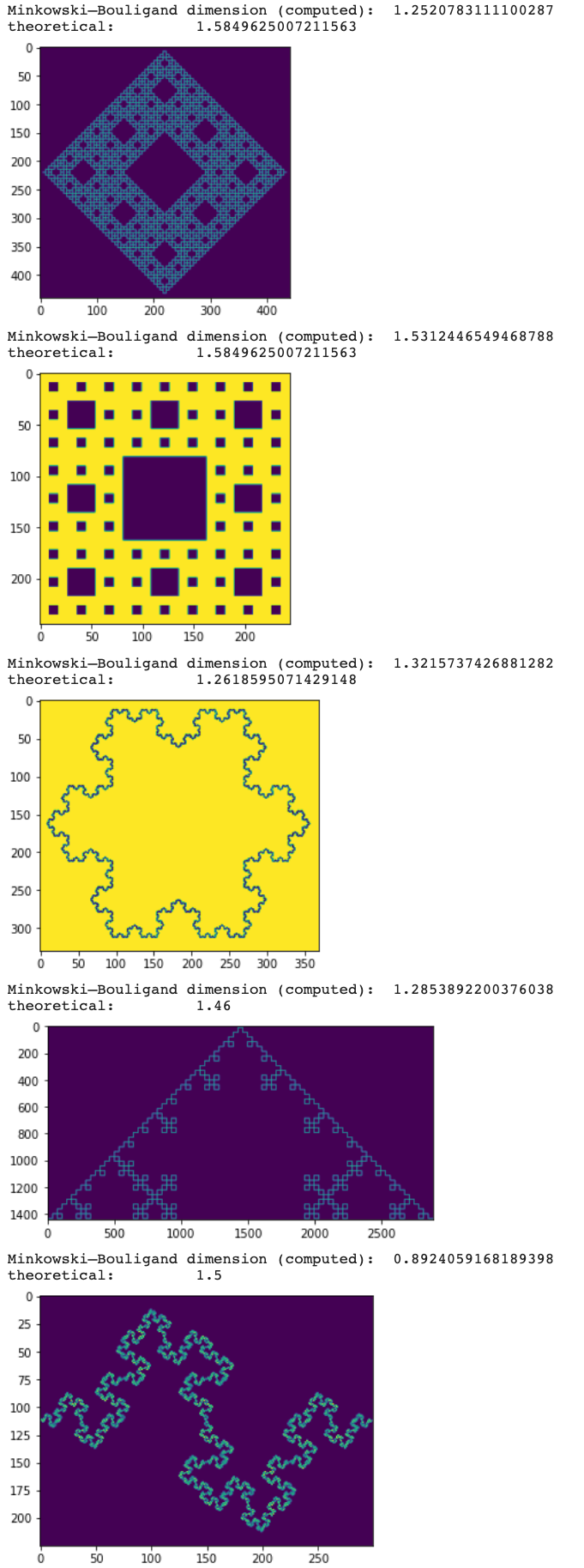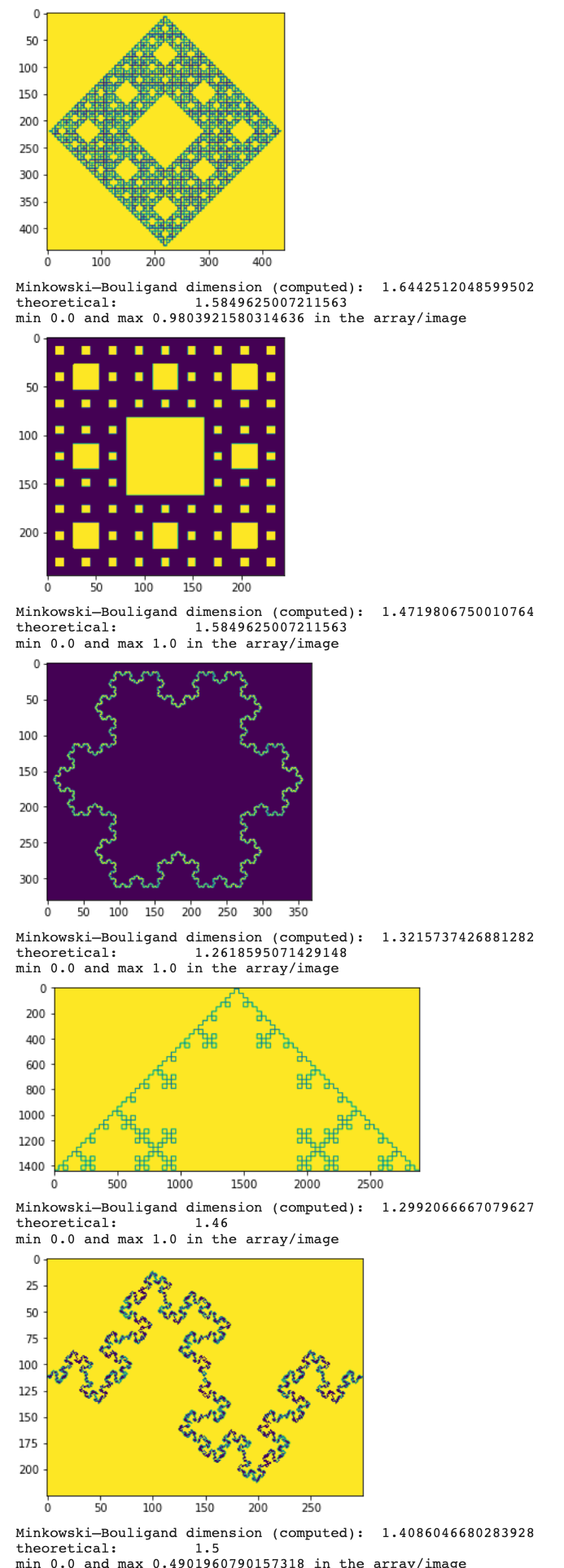{{ message }}

Instantly share code, notes, and snippets.

# rougier/fractal-dimension.py

Last active Oct 15, 2021
Fractal dimension computing
This file contains bidirectional Unicode text that may be interpreted or compiled differently than what appears below. To review, open the file in an editor that reveals hidden Unicode characters. Learn more about bidirectional Unicode characters
 # ----------------------------------------------------------------------------- # From https://en.wikipedia.org/wiki/Minkowski–Bouligand_dimension: # # In fractal geometry, the Minkowski–Bouligand dimension, also known as # Minkowski dimension or box-counting dimension, is a way of determining the # fractal dimension of a set S in a Euclidean space Rn, or more generally in a # metric space (X, d). # ----------------------------------------------------------------------------- import scipy.misc import numpy as np def fractal_dimension(Z, threshold=0.9): # Only for 2d image assert(len(Z.shape) == 2) # From https://github.com/rougier/numpy-100 (#87) def boxcount(Z, k): S = np.add.reduceat( np.add.reduceat(Z, np.arange(0, Z.shape, k), axis=0), np.arange(0, Z.shape, k), axis=1) # We count non-empty (0) and non-full boxes (k*k) return len(np.where((S > 0) & (S < k*k))) # Transform Z into a binary array Z = (Z < threshold) # Minimal dimension of image p = min(Z.shape) # Greatest power of 2 less than or equal to p n = 2**np.floor(np.log(p)/np.log(2)) # Extract the exponent n = int(np.log(n)/np.log(2)) # Build successive box sizes (from 2**n down to 2**1) sizes = 2**np.arange(n, 1, -1) # Actual box counting with decreasing size counts = [] for size in sizes: counts.append(boxcount(Z, size)) # Fit the successive log(sizes) with log (counts) coeffs = np.polyfit(np.log(sizes), np.log(counts), 1) return -coeffs I = scipy.misc.imread("sierpinski.png")/256.0 print("Minkowski–Bouligand dimension (computed): ", fractal_dimension(I)) print("Haussdorf dimension (theoretical): ", (np.log(3)/np.log(2)))

###bhatsrinidhi commented Feb 6, 2018

 Hi, this piece of code is really useful. Please can you help me out, I am having trouble in figuring out the "boxcount" method written. My particular confusion lies in the return statement, "return len(np.where((S > 0) & (S < kk)))" S>0 implies that there is some value in that pixel, but what about the later part i.e. S

###manish-rana001 commented Mar 20, 2018

 Hi there, I would like to know that what is the use of threshold=0.9 value, why we are using this value.

###vansjyo commented Mar 8, 2020

 Better to use `imageio.imread` in line 52 as `imread` is deprecated in SciPy 1.0.0.

###delton137 commented Nov 3, 2020

 Does this code work? I have tried running it on a 2D box generated as follows: ``````seg = np.zeros(shape = (100,100)) seg[20:80,20:80] = 1.0 `````` The function returned 'nan'. I was expecting something close to 2.0. When I ran it on an irregular segmentation, I got fractal dimensions between 0.45 - 0.9. The reference values I found in the literature for the same type of segmentation are around 1.2.

###rougier commented Nov 4, 2020

 Do you have the log (reason for NaN)?

###delton137 commented Nov 5, 2020

 When I run this: `seg = np.zeros(shape = (100,100)) seg[20:80,20:80] = 1.0 fractal_dimension(seg)` In a Jupyter notebook I get "RuntimeWarning: divide by zero encountered in log plt.plot(np.log(sizes), np.log(counts))" It seems there is a problem with trying to fit nearly linear data.

###delton137 commented Nov 5, 2020

 Hi, I looked at this a bit more today. the problem is one of the "counts" is 0 so log(counts) = -inf : counts = [1.38629436 2.7080502 2.99573227 3.68887945 -inf] I can remove the -inf term using this: ``````x = np.log(sizes) y = np.log(counts) mask = ~np.isinf(y) x = x[mask] y = y[mask] coeffs = np.polyfit(x, y, 1) return -coeffs `````` The result is I get D = 1.038. I was expecting it to be closer to 2.

###rougier commented Nov 6, 2020

 Yes, I would have expected 2. Do you have by any change a reference implementation somewhere such that we can check?

###delton137 commented Nov 6, 2020

 Here is the code I'm using : https://repl.it/repls/AlarmingHarmlessScience Thank you for your help with this!!

###rougier commented Nov 10, 2020

 Looking at https://en.wikipedia.org/wiki/Minkowski%E2%80%93Bouligand_dimension, I think I may have computer the dimension of the border and not the actual shape because I filtered out the full boxes. This may explain why the result is 1.

###delton137 commented Nov 10, 2020

 If I run on a rectangle, I get something around 0.55. I think it should be pretty close to either 1 or 2 depending how you did it. To be honest I haven't had time to review your code in depth. I am actually analyzing a 3D shape and using fractal dimension as a measure of surface irregularity, so I'm using a 3D fractal dimension code I found elsewhere for that. So this is not a high priority for me. However I thought it might be worth looking at the fractal dimension for 2D slices of the object as well.

###akshahi17 commented Jan 19, 2021

 I am getting this error message when executing this programme in PyCharm. 1)"AttributeError: module 'scipy.misc' has no attribute 'imread'" 2) "AttributeError: module 'scipy' has no attribute 'misc'"

###rougier commented Jan 20, 2021

 @akshahi17 Probably the imread function has been deprecated in scipy. You cn now use the imageio library instead.

###jankaWIS commented Mar 16, 2021 • edited Loading

 Hi, I was testing it on some fractals with defined dimensions, below is my code. The problem is that it's far from the dimensions and even for the same fractal in a different rotation (Sherpinski), it is not giving the same values (which I believe should, this should be independent of rotation). Where is the problem? Also, I believe that it should go to squares 2x2, that is: `sizes = 2**np.arange(n, 2, -1)` should become `sizes = 2**np.arange(n, 0, -1)`. https://en.wikipedia.org/wiki/Koch_snowflake ```# ----------------------------------------------------------------------------- # From https://en.wikipedia.org/wiki/Minkowski–Bouligand_dimension: # # In fractal geometry, the Minkowski–Bouligand dimension, also known as # Minkowski dimension or box-counting dimension, is a way of determining the # fractal dimension of a set S in a Euclidean space Rn, or more generally in a # metric space (X, d). # ----------------------------------------------------------------------------- import numpy as np import matplotlib.pyplot as plt def fractal_dimension(Z, threshold=0.9): # Only for 2d image assert(len(Z.shape) == 2) # From https://github.com/rougier/numpy-100 (#87) def boxcount(Z, k): S = np.add.reduceat( np.add.reduceat(Z, np.arange(0, Z.shape, k), axis=0), np.arange(0, Z.shape, k), axis=1) # We count non-empty (0) and non-full boxes (k*k) return len(np.where((S > 0) & (S < k*k))) # Transform Z into a binary array Z = (Z < threshold) # Minimal dimension of image p = min(Z.shape) # Greatest power of 2 less than or equal to p n = 2**np.floor(np.log(p)/np.log(2)) # Extract the exponent n = int(np.log(n)/np.log(2)) # Build successive box sizes (from 2**n down to 2**1) sizes = 2**np.arange(n, 1, -1) # Actual box counting with decreasing size counts = [] for size in sizes: counts.append(boxcount(Z, size)) # Fit the successive log(sizes) with log (counts) coeffs = np.polyfit(np.log(sizes), np.log(counts), 1) return -coeffs special = [ 'https://upload.wikimedia.org/wikipedia/commons/thumb/7/7c/Peano_Sierpinski_carpet_4.svg/440px-Peano_Sierpinski_carpet_4.svg.png', 'https://upload.wikimedia.org/wikipedia/commons/thumb/3/3b/Quadratic_Koch_2.svg/2880px-Quadratic_Koch_2.svg.png', 'https://upload.wikimedia.org/wikipedia/commons/thumb/5/55/Quadratic_Koch.svg/300px-Quadratic_Koch.svg.png' ] images = [ 'https://upload.wikimedia.org/wikipedia/commons/thumb/7/7c/Peano_Sierpinski_carpet_4.svg/440px-Peano_Sierpinski_carpet_4.svg.png', 'https://upload.wikimedia.org/wikipedia/commons/thumb/b/b7/Sierpinski_carpet_3.svg/244px-Sierpinski_carpet_3.svg.png', 'https://upload.wikimedia.org/wikipedia/commons/f/f0/Flocke.PNG', 'https://upload.wikimedia.org/wikipedia/commons/thumb/3/3b/Quadratic_Koch_2.svg/2880px-Quadratic_Koch_2.svg.png', 'https://upload.wikimedia.org/wikipedia/commons/thumb/5/55/Quadratic_Koch.svg/300px-Quadratic_Koch.svg.png' ] dimensions = [(np.log(3)/np.log(2)), (np.log(3)/np.log(2)), (np.log(4)/np.log(3)), 1.46, 1.5] for dim, im in zip(dimensions, images): # load im x = plt.imread(im) if im in special: print("Minkowski–Bouligand dimension (computed): ", fractal_dimension(x[:,:,3])) print("theoretical: ", dim) plt.imshow(x[:,:,3]) plt.show() else: print("Minkowski–Bouligand dimension (computed): ", fractal_dimension(x[:,:,1])) print("theoretical: ", dim) plt.imshow(x[:,:,1]) plt.show()``` and the result: Minkowski–Bouligand dimension (computed): 1.2520783111100287 theoretical: 1.5849625007211563 Minkowski–Bouligand dimension (computed): 1.5312446549468788 theoretical: 1.5849625007211563 Minkowski–Bouligand dimension (computed): 1.3215737426881282 theoretical: 1.2618595071429148 Minkowski–Bouligand dimension (computed): 1.2853892200376038 theoretical: 1.46 Minkowski–Bouligand dimension (computed): 0.8924059168189398 theoretical: 1.5###rougier commented Mar 17, 2021

 I'm not sure but the images seems to be a bit noisy which might impact the computation. Can you try to make black and white (for example `I = I > 0.5` and check if the result is the same? If this is the case, this means we need some threshold value when deciding if a box is empty or not.

###jankaWIS commented Mar 17, 2021 • edited Loading

 Except for the first and last, they are all binary (ie B&W). I have tried and you were right, @rougier, about those, setting the threshold to `0.2` works on them. What about this note? Also, I believe that it should go to squares 2x2, that is: `sizes = 2**np.arange(n, 2, -1)` should become `sizes = 2**np.arange(n, 0, -1)`. I am curious because you used to have it that way (at least that is what I see in the history) but you have a discrepancy in the note and in the code. Your code says: `2**np.arange(n, 1, -1) # eg for n=4 you get array([16, 8, 4])` but the comment says `Build successive box sizes (from 2**n down to 2**1)` which suggest this code: `2**np.arange(n, 0, -1) # eg for n=4 you get array([16, 8, 4, 2])` And that I believe is the correct code. Code ```import numpy as np import matplotlib.pyplot as plt def fractal_dimension(Z, threshold=0.9): # Only for 2d image assert(len(Z.shape) == 2) # From https://github.com/rougier/numpy-100 (#87) def boxcount(Z, k): S = np.add.reduceat( np.add.reduceat(Z, np.arange(0, Z.shape, k), axis=0), np.arange(0, Z.shape, k), axis=1) # We count non-empty (0) and non-full boxes (k*k) return len(np.where((S > 0) & (S < k*k))) # Transform Z into a binary array Z = (Z < threshold) plt.imshow(Z) plt.show() # Minimal dimension of image p = min(Z.shape) # Greatest power of 2 less than or equal to p n = 2**np.floor(np.log(p)/np.log(2)) # Extract the exponent n = int(np.log(n)/np.log(2)) # Build successive box sizes (from 2**n down to 2**1) sizes = 2**np.arange(n, 1, -1) # Actual box counting with decreasing size counts = [] for size in sizes: counts.append(boxcount(Z, size)) # Fit the successive log(sizes) with log (counts) coeffs = np.polyfit(np.log(sizes), np.log(counts), 1) return -coeffs special = [ 'https://upload.wikimedia.org/wikipedia/commons/thumb/7/7c/Peano_Sierpinski_carpet_4.svg/440px-Peano_Sierpinski_carpet_4.svg.png', 'https://upload.wikimedia.org/wikipedia/commons/thumb/3/3b/Quadratic_Koch_2.svg/2880px-Quadratic_Koch_2.svg.png', 'https://upload.wikimedia.org/wikipedia/commons/thumb/5/55/Quadratic_Koch.svg/300px-Quadratic_Koch.svg.png' ] images = [ 'https://upload.wikimedia.org/wikipedia/commons/thumb/7/7c/Peano_Sierpinski_carpet_4.svg/440px-Peano_Sierpinski_carpet_4.svg.png', 'https://upload.wikimedia.org/wikipedia/commons/thumb/b/b7/Sierpinski_carpet_3.svg/244px-Sierpinski_carpet_3.svg.png', 'https://upload.wikimedia.org/wikipedia/commons/f/f0/Flocke.PNG', 'https://upload.wikimedia.org/wikipedia/commons/thumb/3/3b/Quadratic_Koch_2.svg/2880px-Quadratic_Koch_2.svg.png', 'https://upload.wikimedia.org/wikipedia/commons/thumb/5/55/Quadratic_Koch.svg/300px-Quadratic_Koch.svg.png' ] dimensions = [(np.log(3)/np.log(2)), (np.log(3)/np.log(2)), (np.log(4)/np.log(3)), 1.46, 1.5] for dim, im in zip(dimensions, images): # load im x = plt.imread(im) if im in special: print("Minkowski–Bouligand dimension (computed): ", fractal_dimension(x[:,:,3], threshold=0.2)) print("theoretical: ", dim) print(f"min {x[:,:,3].min()} and max {x[:,:,3].max()} in the array/image") else: print("Minkowski–Bouligand dimension (computed): ", fractal_dimension(x[:,:,1],threshold=0.2)) print("theoretical: ", dim) print(f"min {x[:,:,1].min()} and max {x[:,:,1].max()} in the array/image")```###jankaWIS commented Mar 17, 2021

 I am getting this error message when executing this programme in PyCharm. 1)"AttributeError: module 'scipy.misc' has no attribute 'imread'" 2) "AttributeError: module 'scipy' has no attribute 'misc'" @akshahi17 check this answer https://stackoverflow.com/questions/15345790/scipy-misc-module-has-no-attribute-imread the function has been deprecated so you can either downgrade (not recommended) or use what @rougier recommended (`imageio`) or what I'm using, `imread` in `matplotlib.pyplot`.